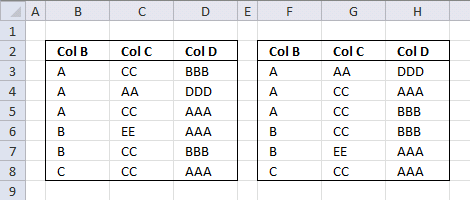Author: Oscar Cronquist Article last updated on August 09, 2017The sorted table to the right is created with an array formula using the data in the table to the left.

Array formula in cell F3:

=INDEX(\$B\$3:\$D\$8, MATCH(SMALL(COUNTIF(\$B\$3:\$B\$8, "<"&\$B\$3:\$B\$8)+COUNTIF(\$C\$3:\$C\$8, "<"&\$C\$3:\$C\$8)/10+COUNTIF(\$D\$3:\$D\$8, "<"&\$D\$3:\$D\$8)/100, ROW(A1)), COUNTIF(\$B\$3:\$B\$8, "<"&\$B\$3:\$B\$8)+COUNTIF(\$C\$3:\$C\$8, "<"&\$C\$3:\$C\$8)/10+COUNTIF(\$D\$3:\$D\$8, "<"&\$D\$3:\$D\$8)/100, 0), COLUMN(A1))

Recommended article

Sort a column alphabetically

Table of Contents Sort a column using array formula Two columns sorting by the second column Sort alphanumeric values I […]

or use this regular formula:

Formula in cell F3:

=INDEX(\$B\$3:\$D\$8, MATCH(SMALL(INDEX(COUNTIF(\$B\$3:\$B\$8, "<"&\$B\$3:\$B\$8)+COUNTIF(\$C\$3:\$C\$8, "<"&\$C\$3:\$C\$8)/10+COUNTIF(\$D\$3:\$D\$8, "<"&\$D\$3:\$D\$8)/100, 0, 0), ROW(B1)), INDEX(COUNTIF(\$B\$3:\$B\$8, "<"&\$B\$3:\$B\$8)+COUNTIF(\$C\$3:\$C\$8, "<"&\$C\$3:\$C\$8)/10+COUNTIF(\$D\$3:\$D\$8, "<"&\$D\$3:\$D\$8)/100, 0, 0), 0), COLUMN(A1))

How to create an array formula

1. Copy array formula
2. Select cell F3
3. Click in formula bar4. Paste array formula to formula bar
5. Press and hold CTRL + SHIFT
6. Press Enter

Recommended article

How to enter an array formula

Array formulas allows you to do advanced calculations not possible with regular formulas.

How to copy array formula

1. Select cell F3
2. Copy cell (Ctrl +c)
3. Select cell range G:3:H3
4. Paste (Ctrl + v)
5. Select cell range F3:H3
6. Copy (Ctrl + c)
7. Select cell range F4:H8
8. Paste (Ctrl + v)

### How does this array formula work?

Step 1 - Sort Col B from A to Z with COUNTIF function

COUNTIF(\$B\$3:\$B\$8, "<"&\$B\$3:\$B\$8)

becomes

COUNTIF({"A";"A";"A";"B";"B";"C"},{"<A";"<A";"<A";"<B";"<B";"<C"})

and returns

{0;0;0;3;3;5}

Recommended article

How to use the COUNTIF function

Counts the number of cells that meet a specific condition.

Step 2 - Sort Col C from A to Z with COUNTIF function

COUNTIF(C3:C8,"<"&C3:C8)/10

becomes

COUNTIF({"CC";"AA";"CC";"EE";"CC";"CC"},"<"&{"CC";"AA";"CC";"EE";"CC";"CC"})/10

and returns

{0.1;0;0.1;0.5;0.1;0.1}

Step 3 - Sort Col D from A to Z with COUNTIF function

COUNTIF(D3:D8,"<"&D3:D8)/100

becomes

COUNTIF({"BBB";"DDD";"AAA";"AAA";"BBB";"AAA"},{"<BBB";"<DDD";"<AAA";"<AAA";"<BBB";"<AAA"})/100

and returns

{0.03;0.05;0;0;0.03;0}

Step 4 - Add all values

COUNTIF(\$B\$3:\$B\$8, "<"&\$B\$3:\$B\$8)+COUNTIF(\$C\$3:\$C\$8, "<"&\$C\$3:\$C\$8)/10+COUNTIF(\$D\$3:\$D\$8, "<"&\$D\$3:\$D\$8)/100

becomes

{0;0;0;3;3;5} + {0.1;0;0.1;0.5;0.1;0.1} + {0.03;0.05;0;0;0.03;0}

and returns

{0.13;0.05;0.1;3.5;3.13;5.1}

Step 5 - Return the k-th smallest value

SMALL(array, k)

SMALL(COUNTIF(\$B\$3:\$B\$8, "<"&\$B\$3:\$B\$8)+COUNTIF(\$C\$3:\$C\$8, "<"&\$C\$3:\$C\$8)/10+COUNTIF(\$D\$3:\$D\$8, "<"&\$D\$3:\$D\$8)/100, ROW(A1))

becomes

SMALL({0.13;0.05;0.1;3.5;3.13;5.1}, ROW(A1))

becomes

SMALL({0.13;0.05;0.1;3.5;3.13;5.1}, 1)

and returns 0.05

Recommended article

How to use the SMALL function

The SMALL function lets you extract a number in a cell range based on how small it is compared to the other numbers in the group.

Step 6 - Find the relative position

MATCH(SMALL(COUNTIF(\$B\$3:\$B\$8, "<"&\$B\$3:\$B\$8)+COUNTIF(\$C\$3:\$C\$8, "<"&\$C\$3:\$C\$8)/10+COUNTIF(\$D\$3:\$D\$8, "<"&\$D\$3:\$D\$8)/100, ROW(A1)), COUNTIF(\$B\$3:\$B\$8, "<"&\$B\$3:\$B\$8)+COUNTIF(\$C\$3:\$C\$8, "<"&\$C\$3:\$C\$8)/10+COUNTIF(\$D\$3:\$D\$8, "<"&\$D\$3:\$D\$8)/100, 0)

becomes

MATCH(0.05, {0.13;0.05;0.1;3.5;3.13;5.1}, 0)

and returns 2.

Recommended article

How to use the MATCH function

Identify the position of a value in an array.

Step 7 - Return the corresponding value

=INDEX(\$B\$3:\$D\$8, MATCH(SMALL(COUNTIF(\$B\$3:\$B\$8, "<"&\$B\$3:\$B\$8)+COUNTIF(\$C\$3:\$C\$8, "<"&\$C\$3:\$C\$8)/10+COUNTIF(\$D\$3:\$D\$8, "<"&\$D\$3:\$D\$8)/100, ROW(A1)), COUNTIF(\$B\$3:\$B\$8, "<"&\$B\$3:\$B\$8)+COUNTIF(\$C\$3:\$C\$8, "<"&\$C\$3:\$C\$8)/10+COUNTIF(\$D\$3:\$D\$8, "<"&\$D\$3:\$D\$8)/100, 0), COLUMN(A1))

becomes

=INDEX(\$B\$3:\$D\$8, 2, COLUMN(A1))

becomes

=INDEX(\$B\$3:\$D\$8, 2, 1)

becomes

=INDEX({"A";"A";"A";"B";"B";"C"}, 2, 1)

and returns A in cell F3.

Recommended article

How to use the INDEX function

Gets a value in a specific cell range based on a row and column number.